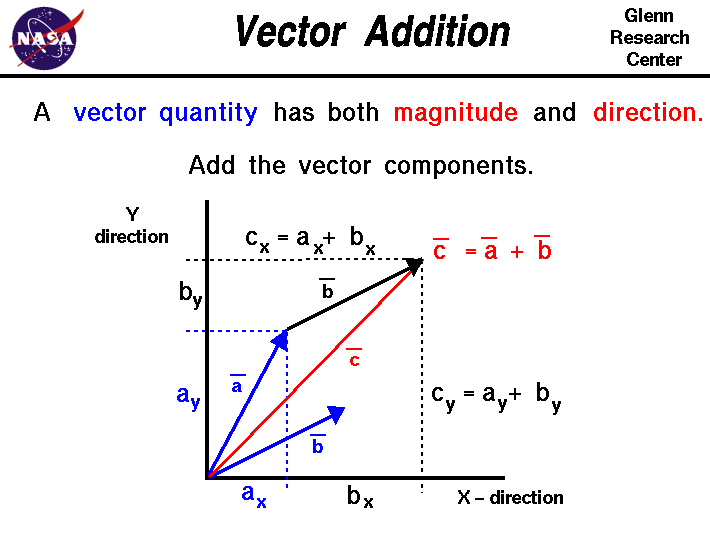+ Text Only Site
+ Non-Flash Version
+ Contact GlennMath and science were invented by humans to describe and understand the world around us. We observe that there are some quantities and processes in our world that depend on the direction in which they occur, and there are some quantities that do not depend on direction. Mathematicians and scientists call a quantity which depends on direction a vector quantity. A quantity which does not depend on direction is called a scalar quantity. A vector quantity has two characteristics, a magnitude and a direction. When comparing two vector quantities of the same type, you have to compare both the magnitude and the direction. On this slide we describe the method for adding two vectors. Vector addition is one aspect of a larger vector algebra which we are not going to present at this web site. Vector addition is presented here because it occurs quite often in the study of propulsion and because it demonstrates some fundamental differences between vectors and scalars. Vectors are usually denoted on figures by an arrow. The length of the arrow indicates the magnitude and the tip of the arrow indicates the direction. The vector is labeled with an alphabetical letter with a line over the top to distinguish it from a scalar. We will denote the magnitude of the vector by the symbol |a|. The direction will be measured by an angle phi relative to a coordinate axis x. The coordinate axis y is perpendicular to x. Note: The coordinate axes x and y are themselves vectors! They have a magnitude and a direction. You first encounter coordinates axes when you learn to graph. So, you have been using vectors for some time without even knowing it! If we construct a dashed line from the tip of the vector a running parallel to the x-axis, it cuts the y-axis at a location we label ay. Similarly, a line from the tip of the vector parallel to the y-axis cuts the x-axis at ax. The quanities ax and ay are called the components of the vector and both are scalar quantites. To add two vectors, a and b, we first break each vector into its components, ax and ay, and bx and by, as shown on the figure. From the rules which govern the equality of vectors, the blue vector b is equal to the black vector b because it has equal equal length and equal direction. Now since the components of vector a and vector b are scalars, we can add the x-components to generate the x-component of new vector c: cx = ax + bx Similarly, we can add the y-components: cy = ay + by The new components, cx and cy, completely define the new vector c by specifying both the magnitude and the direction. Looking carefully at the diagram, we see that adding two vectors produces a new vector which is not in the direction of either of the original vectors, and whose magnitude is not equal to the sum of the magnitudes of the original vectors. Vector algebra is very different from scalar algebra because it must account for both magnitude and direction. Note: On this slide, for simplicity, we have developed the components in only two dimensions; there are two coordinate axes. In reality, there are three spatial dimensions and three components of all forces. This is important in our derivation of the general equations of with motion for flight trajectories and for the Navier-Stokes and Euler equations which describe the forces and resulting motion of fluids in the engine. We can break very complex, three-dimensional, vector problems into only three scalar equations. Activities: Guided Tours Navigation ..Beginner's Guide Home Page+ Inspector General Hotline + Equal Employment Opportunity Data Posted Pursuant to the No Fear Act + Budgets, Strategic Plans and Accountability Reports + Freedom of Information Act + The President's Management Agenda + NASA Privacy Statement, Disclaimer, and Accessibility CertificationEditor: Nancy Hall NASA Official: Nancy Hall Last Updated: May 05 2015 + Contact Glenn